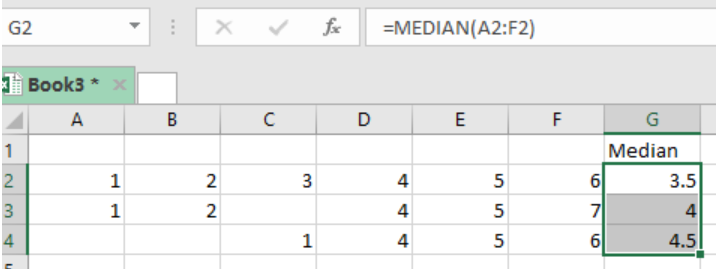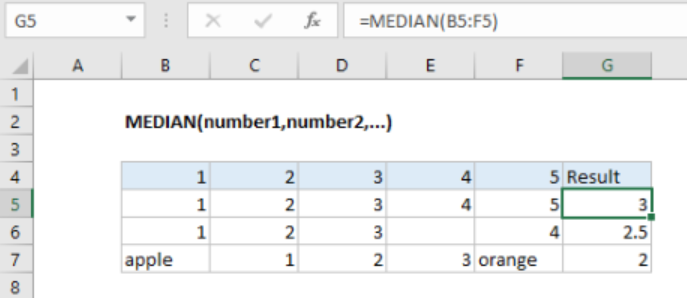Get instant live expert help with Excel or Google Sheets“My Excelchat expert helped me in less than 20 minutes, saving me what would have been 5 hours of work!”

#### Post your problem and you’ll get expert help in seconds.

Your message must be at least 40 characters
Our professional experts are available now. Your privacy is guaranteed.

# Pro Tips on How to Find the Median in Excel

We can now find the median or middle number of a group of supplied numbers in an easy and straightforward way in excel. To learn how to do this, read this post to the end.Figure 1: How to find median in excel

## General syntax of the formula

`=MEDIAN (number1, [number2],…)`

Where;

• Number1- this is the number of cell reference referring to the numeric values
• Number2- this is the number or cell reference that refers to the optional numeric values

## How the formula works

• The formula will return the middle value as the median if the total number supplied is odd.
• The formula will find the average of the two middle numbers of the total numbers supplied is even.
• Those cells with text, logic values or no values will be ignored by the formula
• The formula accept numbers in various forms including numbers, ranges, named ranges as well as cell references.
• The formula can accept up to 255 numbers at any given one time.

## ExampleFigure 2: Example of how to find median in excel

In this example, we want to find the median of the values in each of the rows.

Step 1: Fill your data in the sheet from cell B4 to cell G7 as shown above. The “Result” column shall have the median values of the rows.

Step 2: In cell G5, enter the formula `=MEDIAN(B5:F5)`

Step 3: Press Enter to get the median

Step 4: Copy down the formula across the other cells to get the median for the other rows.

## Instant Connection to an Expert through our Excelchat Service

Most of the time, the problem you will need to solve will be more complex than a simple application of a formula or function. If you want to save hours of research and frustration, try our live Excelchat service! Our Excel Experts are available 24/7 to answer any Excel question you may have. We guarantee a connection within 30 seconds and a customized solution within 20 minutes.

Solution examplesNeed to calculate out two equally spaced points between a range of dates. Basically like the MEDIAN function between two cells that have dates, but I need to produce two equally spaced points between that range. For example. If I have the following date range (A1) 2/2/2018 (B1) 7/2/2018, then that's roughly 150 days total. I want 4 equally spaced points (Start, Start-Mid, Mid-End, End). So I'd say that's 50 day chunks spaced euqally. Which would produce 4 total dates 2/2/2018 - 3/24/2018 - 5/13/2018 - 7/2/2018.
Solved by K. J. in 20 minsi have this formula currently which works but not if I want it for every day - any ideas ?? (NETWORKDAYS.INTL(L1362,M1362,11,BH!A:A)-1)*("17:30"-"8:30")+IF(NETWORKDAYS.INTL(M1362,M1362,11,BH!A:A),MEDIAN(MOD(M1362,1),"8:30","17:30"),"17:30")-MEDIAN(NETWORKDAYS.INTL(L1362,L1362,11,BH!A:A)*MOD(L1362,1),"8:30","17:30")
Solved by A. D. in 28 mins